Courses

# Constructional Features Of DC Machines Electrical Engineering (EE) Notes | EduRev

## Electrical Engineering (EE) : Constructional Features Of DC Machines Electrical Engineering (EE) Notes | EduRev

The document Constructional Features Of DC Machines Electrical Engineering (EE) Notes | EduRev is a part of the Electrical Engineering (EE) Course Electrical Machines.
All you need of Electrical Engineering (EE) at this link: Electrical Engineering (EE)

Constructional Features of DC Machines
(Refer Slide Time: 00:29)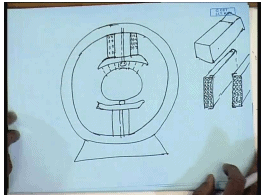In the last lecture we have seen, one of the main constructional feature of a DC machine is a stator cylinder. So, if we take a cross section of that, some risk this is made up of iron. Now, this iron is usually solid, because it carries DC flux. So, there is no need to laminate this, and these iron bodies the field course, which are produce separately are bolded down, the poles themselves are rectangular pieces of irons, again normally they carry DC flux. So, not laminated as such the body is not laminated.

So, this is the pole structure, which carries the field winding, field winding is made on a separate cylinder, and depending on what type of winding it is, that is either shunt or series, the number turns and the current carrying capacity of the turns are determined and showing a winding for shunt connection or separate excitation, which are normally made up of thin wires of large number of turns, this winding again a very queues in length. So, that can be placed on this field.

So, this winding is placed on the field. So, from outside it will look like, in a cross section it look like a body like this, on which this field winding is placed, and this hole assembly is kept placed together by set of pole shoes, the hole arrangement is then along with the pole shoe is bolted to the stator core, this is the construction of one pole there may similarly a second pole, poles as you known comes in pairs.

The purpose of the pole shoe is not only to hold the field coil and the field pole together, but also to adjust the air gap, between the armature and the stator pole. So, that the flux density on the armature surface, can be given a desired wave shape, usually this flux density is trapezoidal in nature. Now, although the main pole body or the back side core of the stator and not laminated, this pole shoe are usually laminated.

The reason can be understood from this diagram, in order to accommodate winding, the armature surface is usually slotted, therefore the reluctance between the pole shoe surface and the armature is not constant, it varies as there are alternate tooth’s and slots on the armature surface, therefore for weak DC excitation on the hint coil, the flux density in the field pole will also see variation in the same frequency as the occurrence of the tooth and slots in the rotor.

So, the pole shoes experiences a flux density waveform, which has a high frequency alternating component depending on the number of slots on the armature surface and its rotational speed. So, there is an alternating flux in the pole shoes, in order to prevent a larger amount eddy current loss due this alternating flux, normally the post pole shoes are laminated. This is one of the salient point about the stator pole arrangement, as we have mentioned earlier, the stator may have more than two pole pairs all of them normally identical, the field windings may be either supplied from a separate source or they may be connected across the armature or in series with the armature giving different possibilities of separately excited, shunt excited and series excited or even compound excitated machine.

However, the place where a DC machine differs significantly from other types of machine in its armature construction, we have seen that, one predominate feature of the DC machine armature is that it carries a commuter, which is necessary in order to change the direction of current flowing through individual coils in a DC machine armature.

The armature of a DC machine also differs from the armature winding of other electrical AC electrical machines in the sense, but this is a closed winding, that is the finish of the winding and the start of the winding are shorted together, we have also explained in the last class, in the last lecture that there can be two types of winding in a DC machine, one is called the lap winding and another is called the wave winding, just look at the difference between the two for that

(Refer Slide Time: 10:52)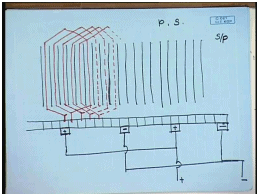Let us say, we have a machine with several slots, this is one slot, second, third, fourth these are the different slots in this DC machine. Similarly, there are commutated segments, normally we have seen that in a DC machine the number of commutated segment should be equal to the number of coils, a DC machine, a coil is made up of two coils sides, which are placed in two different slots.

However, DC machines are usually for with what is called the double layer winding that is each slot contains two coils sides, one on the top and another in the bottom. So, number of slots is equal to the number of coils and hence, number of commutated segment is also equal to the number of slots. So, let us say these said commutated segments separated by insulators.

The coils are placed inside the slots. This is one coil, say this is another coil said, inside these are the coil sides connecting two coil sides. So, a lap winding progress like this, coil side is connected to this commutated segment, the other coil side is connects to this commutated segment, third coil goes to the next, this is how a lap winding progresses and finally, the in coil will some here and it will produce what is called a closed winding. So, we see that in a lap winding between two consecutive commutated segments, there are there is only one coil, and if one place, one brush here, we have said, if the number of poles of the machine is p and number of slots are s, then there are s number of commutates also and between and number of commutated segment per pole will be s /p. So, after s by p the corresponding other commutated will be there.

The number of coils are also s/s, hence between s by p, there will be 1 by p, number of coils are connected between a positive and a negative set of brushes. So, in a lap winding how many brushes will be needed, that will be equal to the number of poles and between two pairs of brushes, will be same number of coils and which will generate the same voltage. So, this coils can be connected this brushes can be connected in parallelSo, this two plus brush will be connected in parallel, similarly two minus brush will be connected in parallel, to give the two armature terminals, the plus terminal this is the minus terminal

(Refer Slide Time: 18:53)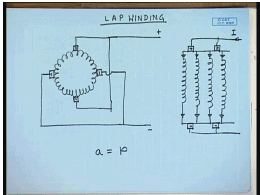So, if we look at the lap winding, the picture arises somewhat like this, this is the closed armature winding, there will be with a four pole construction, there will be four brushes and the connection will be this two will be connected in parallel, similarly this two will be connected in parallel, and finally, this will be the two terminals of the armature.

So, this is a four pole machine we have mentioned earlier, that if the stator number of poles are four then the armature must also be ount for four poles. So, the total set of armature coils can be divided into four groups, each ount for one pole a north, a south, a north, and another south. So, this can be pictorially represented by give coils.

So, there are four per the total armature winding is divided into four equal parts, each part is ount to give a particular pole, two of this poles connects to one positive brush and two connects to the other positive brush, two of this connects to one negative brush and two of this connects to another negative brush, this is the final circuit.

So, if there is a positive current I flowing into this armature, it is is four different paths to flow and that is why, in a lap winding the number of parallel paths, which is denoted that is by a is always equal to the number of poles p of the machines, this is the characteristic of a lap winding, it is easy to understand, that in a lap winding one pole pair each pole pair are connected in parallel, therefore it is suitable for those machines, whose armature voltage rating is small, but the current rating is large, because in this winding number of parallel paths are more with larger number of poles and hence, the total armature current is divided into more number of paths, each path carrying only 1 by p times the total armature current.

(Refer Slide Time: 23:44)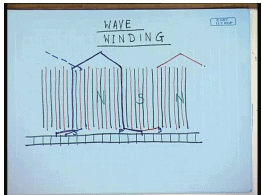Therefore this winding is suitable for DC machines that have large current rating, but less voltage rating. The other type of winding is called the wave winding. For this, let us again consider the slots and also the commutated segments, the arrangement of the coils are coils sides are same the conductors.

However, the difference is in this case, let us say this is the start end of one coil this is the finish end of another coil this possibly produces a north pole here, this connects to the start end of the north pole of the next pole pair. The difference between this gives south pole here, connects to the north pole of the next pole pair, that is a coil goes somewhat like this, this is the start of first coil, this is the finish of first coil, this is the start of the second coil.

The finish of this coil for a four pole machine will come here, and this will connect to this position. So, in this case we see, that between two adjacent commutated commutator coils, there are p by 2 number of between two adjacent commutated segments there are p by 2 number of coils connected, where p is the number of poles, if there are four poles between two adjacent commutated segment there are two coils connected. So, this shows that in essence this wave winding all the field coils, all the armature coils are north pole and south pole all the pole pairs are connected in series. So, equivalently the armature winding of a wave connected machine will look like.

(Refer Slide Time: 29:15)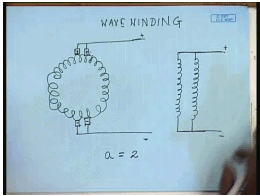This the two positive ends will come here and the two negative brushes will connect to next two coils, this will be the equivalent connection of a wave connected machine, in the equivalent circuit, it will give raised to two parallel personally. It is easily understood that between two adjacent commutated segments there are p by 2 number of coils connected and the opposite commutated segments are 1 by p coils apart, opposite brushes are 1 by p commutated segments apart.

So, between two opposite polarity brushes there are p by 2 into 1 by p that is half the total number of coils are present, between any two opposite polarity brushes. So, half the coil is connected between one set of brush and the rest half is connected between another set of brush, these are shorted and hence, a wave connected machine the number of parallel paths a equal 2. So, we see that while in a lap connected in an armature winding the number of coils between any two opposite polarity brushes is 1 by p, where p is the number of poles in a wave connected machine. The number of parallel paths between two opposite polarity brushes is half of the total number of coils.

So, the number of parallel paths in a lap winding was equal to p, while in a wave connected wave winding. The number of parallel paths is always equal to two. Therefore, we can conclude that a DC machine that has lower voltage rating, but higher current rating. A lap winding should be used, while in a DC machine there are higher voltage rating, but lower current rating, a wave winding will be preferable. Now, let us have a closer loop on the commutated segment itself, if we look at the DC machine, we have seen the cross section

(Refer Slide Time: 33:21)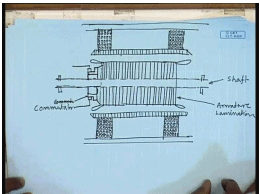Now, if we see the other side view. It will look somewhat like this, the armature shaft, on which the armature is placed, which incidentally laminated, because the voltage induced in the armature is alternating in nature. The current individual coils are also alternating. So, the armature is laminated at the armature coils on the stator body, you have the pole shoe running all along, which is also laminated, this is the shaft, this is the armature body, this is carried on pole pieces, these are the wind windings, this is the stator back iron, on one of this sides the commutators are placed on a separate annolervaing. Commutors have two parts, one part is the sliding surface, on which the brushes slide, the other part is called raiser, to which part the armature conductors are connected. This is the commutated the brushes are connected in this region, this is the brushes, and this is commutated. The shaft is off course carried on bearings on both ends.

(Refer Slide Time: 39:49)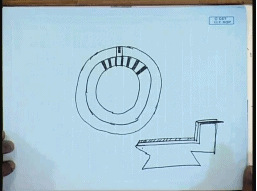So, this is how the side view of a DC machine will look like. Now, if we take a closer look at the commutator itself. The commutator ring is look, somewhat like this. A commutator is made up of copper segments isolated by the raiser part there is group here, where the rectangular armature conductor comes and connects, the commutatos are themselves. Copper segments separated by insulating mica and as we have mentioned earlier, the number of commuatators segments are equal to the number of coils in a DC machine.

A individual commutator segment is a wedge shaft copper with a raiser section, this is an individual commutator segment, this is the raiser part, this is the place, where the armature conductors comes and connects and these kind of segments are alternately placed one commutator segment and then a insulating material and then another commutator segment to form a cylinder, and this hole assembly is then lent on the same shaft as the armature. The brushes are carbon brushes and spring loaded.

So, that they maintain a good contact and the graphite brushes they provide for good lubrications. So, that the commutation can be smooth, in addition to this major constructional feature there are few other constructional features that are important for good performance of the DC machine.

(Refer Slide Time: 43:53)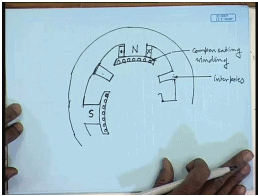These are called one of them is called the inter pole. So, this is the stator of the DC machine, let us say, it is a four pole DC machine. Then there will be one main pole, pair of main pole two pairs of main pole, this is the pole shoe and this is the field winding then, this is let us say the other pair, this is let us say north pole, the other part of that pole pair, this is a south pole, in between these a short in prodding pole structure is introduced, between each of this pole pairs. This is called an inter pole. The requirement of this inert pole is to eight in commutation, we have seen that the brushes connects to winding, whose coil sides are at inter polar location ideally, the voltage induced in this coils should be zero.

However, because of current flowing in the armature, the coil undergoing commutation does not have an induced voltage of zero, because the armature the flux due to the armature current, which is called armature reaction. Distorts the field flux in the inter polar region. So, there will be a net voltage induced in the coil undergoing commutation, which may gives raise to dangerous parks and over currents in order to neutralize the flux in the inter polar region. An inter pole with the requisite number of turns and connected in series with the armature is introduced.

So, that it neutralizes the distortion of field flux due to armature current in the inter polar region. So, this is the purpose of inter pole in addition to inter poles there is another winding, which is placed in series with the armature. It is to be understood that, due to armature current flowing the distortion in field flux occurs not only in the inter polar region, but also in the polar that is under the pole, also there will be some distortion in order to compensate for that, we put what is called set of pole phased conductors? So, that it compensates for the in the main filed flux due to the current flowing in the armature. This flux is also connected, this coils are also connected, in series with the armature, this is called the compensating winding.

(Refer Slide Time: 49:29)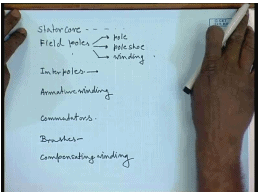So, to summarize we can conclude that the main constructional features of a DC motor are a stator core then field poles, which has several parts one is the pole, pole shoe and winding. Then inter poles, armature winding, then commutators, brushes and compensating winding. These are the primary electrical components in a standard direct current machine and are to be found in one form or other in most of the DC machines.

The purpose of this items are the stator core provides a return path for the filed flux filed pole produces the field flux inter poles and necessary, in order to compensate for the distortion in field flux due to armature current in the inter polar region, then armature winding carries the current, voltages induced in this winding and is required for generating the e m f or producing the torque commutataors provide for direction of current change, which is necessary for the operating proper operation of a DC machine.

Brushes provide moving contact to the commutators and hence, to the armature winding again a compensating winding, which accounts for, which compensates for the distortion in the field flux due to armature current, in the polar region. So, we will in the next lecture few classes, we will discuss how and what exactly will be the expression for induced voltage and taught in a direct current machine, we will also find out, why, how to calculate the ampere turns, for the inter poles and compensating windings.

Offer running on EduRev: Apply code STAYHOME200 to get INR 200 off on our premium plan EduRev Infinity!

61 docs|20 tests

,

,

,

,

,

,

,

,

,

,

,

,

,

,

,

,

,

,

,

,

,

;